# fit

Fit curve or surface to data

## Syntax

``fitobject = fit(x,y,fitType)``
``fitobject = fit([x,y],z,fitType)``
``fitobject = fit(x,y,fitType,fitOptions)``
``fitobject = fit(x,y,fitType,Name,Value)``
``````[fitobject,gof] = fit(x,y,fitType)``````
``````[fitobject,gof,output] = fit(x,y,fitType)``````

## Description

example

````fitobject = fit(x,y,fitType)` creates the fit to the data in `x` and `y` with the model specified by `fitType`.```

example

````fitobject = fit([x,y],z,fitType)` creates a surface fit to the data in vectors `x`, `y`, and `z`.```

example

````fitobject = fit(x,y,fitType,fitOptions)` creates a fit to the data using the algorithm options specified by the `fitOptions` object.```

example

````fitobject = fit(x,y,fitType,Name,Value)` creates a fit to the data using the library model `fitType` with additional options specified by one or more `Name,Value` pair arguments. Use `fitoptions` to display available property names and default values for the specific library model.```

example

``````[fitobject,gof] = fit(x,y,fitType)``` returns goodness-of-fit statistics in the structure `gof`.```

example

``````[fitobject,gof,output] = fit(x,y,fitType)``` returns fitting algorithm information in the structure `output`. ```

## Examples

collapse all

Load some data, fit a quadratic curve to variables `cdate` and `pop`, and plot the fit and data.

```load census; f=fit(cdate,pop,'poly2')```
```f = Linear model Poly2: f(x) = p1*x^2 + p2*x + p3 Coefficients (with 95% confidence bounds): p1 = 0.006541 (0.006124, 0.006958) p2 = -23.51 (-25.09, -21.93) p3 = 2.113e+04 (1.964e+04, 2.262e+04) ```
`plot(f,cdate,pop)`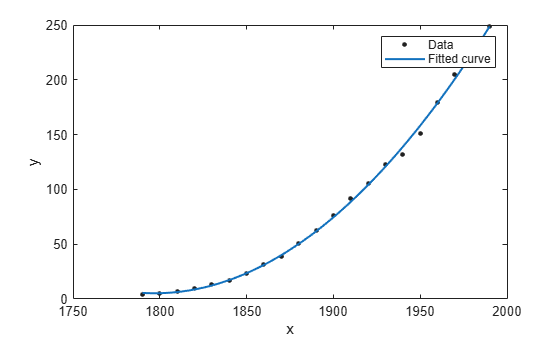For a list of library model names, see `fitType`.

Load some data and fit a polynomial surface of degree 2 in `x` and degree 3 in `y`. Plot the fit and data.

```load franke sf = fit([x, y],z,'poly23')```
``` Linear model Poly23: sf(x,y) = p00 + p10*x + p01*y + p20*x^2 + p11*x*y + p02*y^2 + p21*x^2*y + p12*x*y^2 + p03*y^3 Coefficients (with 95% confidence bounds): p00 = 1.118 (0.9149, 1.321) p10 = -0.0002941 (-0.000502, -8.623e-05) p01 = 1.533 (0.7032, 2.364) p20 = -1.966e-08 (-7.084e-08, 3.152e-08) p11 = 0.0003427 (-0.0001009, 0.0007863) p02 = -6.951 (-8.421, -5.481) p21 = 9.563e-08 (6.276e-09, 1.85e-07) p12 = -0.0004401 (-0.0007082, -0.0001721) p03 = 4.999 (4.082, 5.917) ```
`plot(sf,[x,y],z)`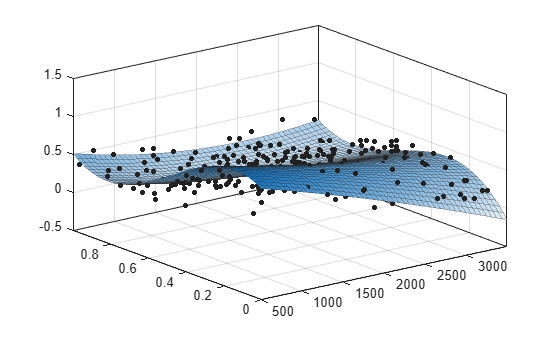Load the `franke` data and convert it to a MATLAB® table.

```load franke T = table(x,y,z);```

Specify the variables in the table as inputs to the `fit` function, and plot the fit.

```f = fit([T.x, T.y],T.z,'linearinterp'); plot( f, [T.x, T.y], T.z )```Load and plot the data, create fit options and fit type using the `fittype` and `fitoptions` functions, then create and plot the fit.

Load and plot the data in `census.mat`.

```load census plot(cdate,pop,'o')```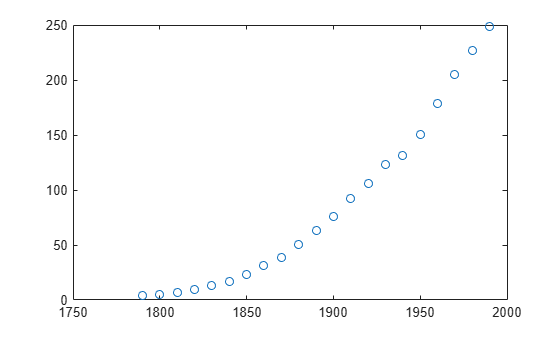Create a fit options object and a fit type for the custom nonlinear model $y=a\left(x-b{\right)}^{n}$, where a and b are coefficients and n is a problem-dependent parameter.

```fo = fitoptions('Method','NonlinearLeastSquares',... 'Lower',[0,0],... 'Upper',[Inf,max(cdate)],... 'StartPoint',[1 1]); ft = fittype('a*(x-b)^n','problem','n','options',fo);```

Fit the data using the fit options and a value of n = 2.

`[curve2,gof2] = fit(cdate,pop,ft,'problem',2)`
```curve2 = General model: curve2(x) = a*(x-b)^n Coefficients (with 95% confidence bounds): a = 0.006092 (0.005743, 0.006441) b = 1789 (1784, 1793) Problem parameters: n = 2 ```
```gof2 = struct with fields: sse: 246.1543 rsquare: 0.9980 dfe: 19 adjrsquare: 0.9979 rmse: 3.5994 ```

Fit the data using the fit options and a value of n = 3.

`[curve3,gof3] = fit(cdate,pop,ft,'problem',3)`
```curve3 = General model: curve3(x) = a*(x-b)^n Coefficients (with 95% confidence bounds): a = 1.359e-05 (1.245e-05, 1.474e-05) b = 1725 (1718, 1731) Problem parameters: n = 3 ```
```gof3 = struct with fields: sse: 232.0058 rsquare: 0.9981 dfe: 19 adjrsquare: 0.9980 rmse: 3.4944 ```

Plot the fit results with the data.

```hold on plot(curve2,'m') plot(curve3,'c') legend('Data','n=2','n=3') hold off```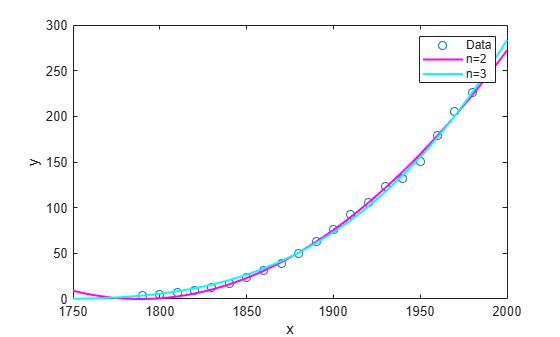Load some data and fit and plot a cubic polynomial with center and scale (`Normalize`) and robust fitting options.

```load census; f=fit(cdate,pop,'poly3','Normalize','on','Robust','Bisquare')```
```f = Linear model Poly3: f(x) = p1*x^3 + p2*x^2 + p3*x + p4 where x is normalized by mean 1890 and std 62.05 Coefficients (with 95% confidence bounds): p1 = -0.4619 (-1.895, 0.9707) p2 = 25.01 (23.79, 26.22) p3 = 77.03 (74.37, 79.7) p4 = 62.81 (61.26, 64.37) ```
`plot(f,cdate,pop)`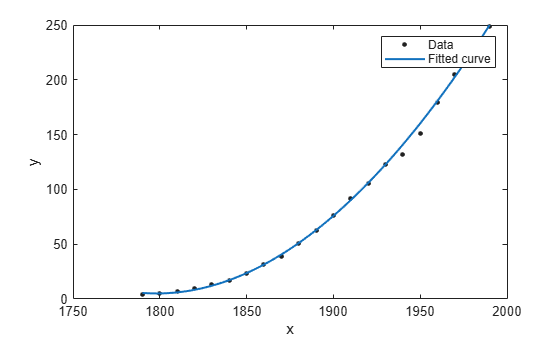Define a function in a file and use it to create a fit type and fit a curve.

Define a function in a MATLAB® file.

```function y = piecewiseLine(x,a,b,c,d,k) % PIECEWISELINE A line made of two pieces % that is not continuous. y = zeros(size(x)); % This example includes a for-loop and if statement % purely for example purposes. for i = 1:length(x) if x(i) < k, y(i) = a + b.* x(i); else y(i) = c + d.* x(i); end end end```

Save the file.

Define some data, create a fit type specifying the function `piecewiseLine`, create a fit using the fit type `ft`, and plot the results.

```x = [0.81;0.91;0.13;0.91;0.63;0.098;0.28;0.55;... 0.96;0.96;0.16;0.97;0.96]; y = [0.17;0.12;0.16;0.0035;0.37;0.082;0.34;0.56;... 0.15;-0.046;0.17;-0.091;-0.071]; ft = fittype( 'piecewiseLine( x, a, b, c, d, k )' ) f = fit( x, y, ft, 'StartPoint', [1, 0, 1, 0, 0.5] ) plot( f, x, y ) ```

Load some data and fit a custom equation specifying points to exclude. Plot the results.

Load data and define a custom equation and some start points.

```[x, y] = titanium; gaussEqn = 'a*exp(-((x-b)/c)^2)+d'```
```gaussEqn = 'a*exp(-((x-b)/c)^2)+d' ```
`startPoints = [1.5 900 10 0.6]`
```startPoints = 1×4 1.5000 900.0000 10.0000 0.6000 ```

Create two fits using the custom equation and start points, and define two different sets of excluded points, using an index vector and an expression. Use `Exclude` to remove outliers from your fit.

`f1 = fit(x',y',gaussEqn,'Start', startPoints, 'Exclude', [1 10 25])`
```f1 = General model: f1(x) = a*exp(-((x-b)/c)^2)+d Coefficients (with 95% confidence bounds): a = 1.493 (1.432, 1.554) b = 897.4 (896.5, 898.3) c = 27.9 (26.55, 29.25) d = 0.6519 (0.6367, 0.6672) ```
`f2 = fit(x',y',gaussEqn,'Start', startPoints, 'Exclude', x < 800)`
```f2 = General model: f2(x) = a*exp(-((x-b)/c)^2)+d Coefficients (with 95% confidence bounds): a = 1.494 (1.41, 1.578) b = 897.4 (896.2, 898.7) c = 28.15 (26.22, 30.09) d = 0.6466 (0.6169, 0.6764) ```

Plot both fits.

```plot(f1,x,y) title('Fit with data points 1, 10, and 25 excluded')``````figure plot(f2,x,y) title('Fit with data points excluded such that x < 800')```You can define the excluded points as variables before supplying them as inputs to the fit function. The following steps recreate the fits in the previous example and allow you to plot the excluded points as well as the data and the fit.

Load data and define a custom equation and some start points.

```[x, y] = titanium; gaussEqn = 'a*exp(-((x-b)/c)^2)+d'```
```gaussEqn = 'a*exp(-((x-b)/c)^2)+d' ```
`startPoints = [1.5 900 10 0.6]`
```startPoints = 1×4 1.5000 900.0000 10.0000 0.6000 ```

Define two sets of points to exclude, using an index vector and an expression.

```exclude1 = [1 10 25]; exclude2 = x < 800;```

Create two fits using the custom equation, startpoints, and the two different excluded points.

```f1 = fit(x',y',gaussEqn,'Start', startPoints, 'Exclude', exclude1); f2 = fit(x',y',gaussEqn,'Start', startPoints, 'Exclude', exclude2);```

Plot both fits and highlight the excluded data.

```plot(f1,x,y,exclude1) title('Fit with data points 1, 10, and 25 excluded')```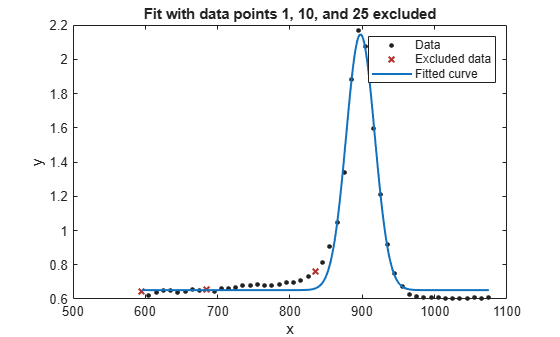```figure; plot(f2,x,y,exclude2) title('Fit with data points excluded such that x < 800')```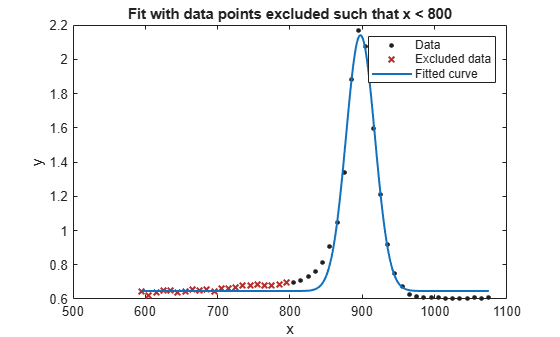For a surface fitting example with excluded points, load some surface data and create and plot fits specifying excluded data.

```load franke f1 = fit([x y],z,'poly23', 'Exclude', [1 10 25]); f2 = fit([x y],z,'poly23', 'Exclude', z > 1); figure plot(f1, [x y], z, 'Exclude', [1 10 25]); title('Fit with data points 1, 10, and 25 excluded')```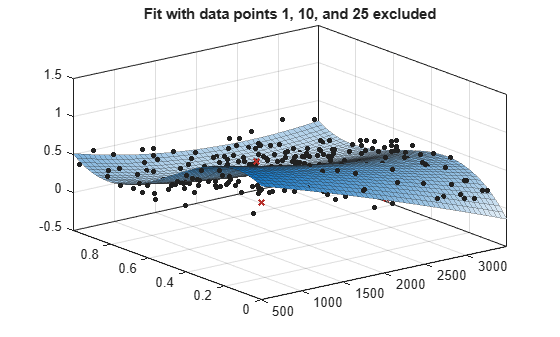```figure plot(f2, [x y], z, 'Exclude', z > 1); title('Fit with data points excluded such that z > 1')```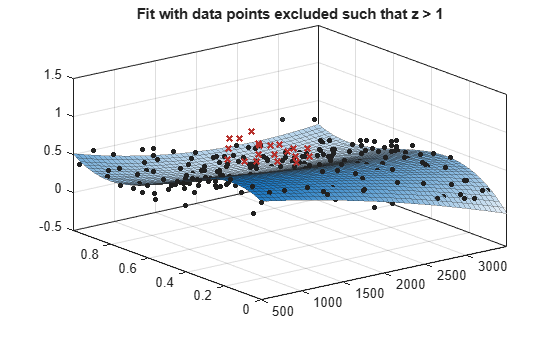Load some data and fit a smoothing spline curve through variables `month` and `pressure`, and return goodness of fit information and the output structure. Plot the fit and the residuals against the data.

```load enso; [curve, goodness, output] = fit(month,pressure,'smoothingspline'); plot(curve,month,pressure); xlabel('Month'); ylabel('Pressure');```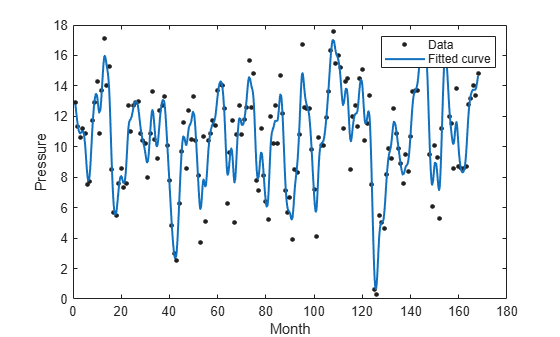Plot the residuals against the x-data (`month`).

```plot( curve, month, pressure, 'residuals' ) xlabel( 'Month' ) ylabel( 'Residuals' )```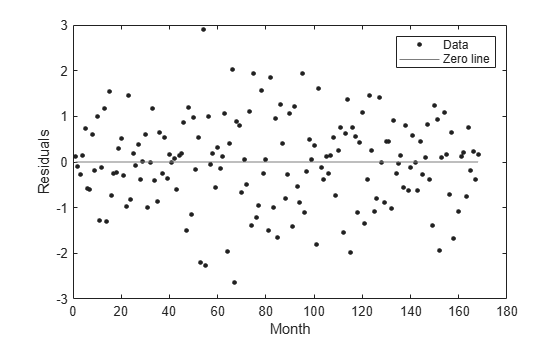Use the data in the `output` structure to plot the residuals against the y-data (`pressure`).

```plot( pressure, output.residuals, '.' ) xlabel( 'Pressure' ) ylabel( 'Residuals' )```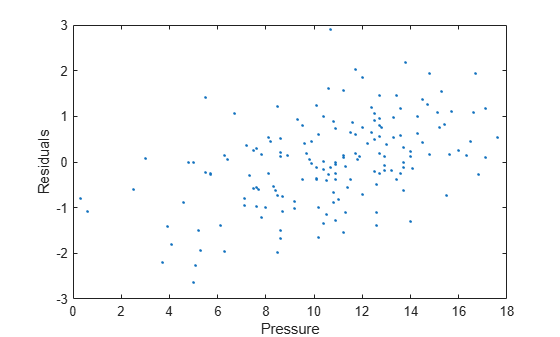Generate data with an exponential trend, and then fit the data using the first equation in the curve fitting library of exponential models (a single-term exponential). Plot the results.

```x = (0:0.2:5)'; y = 2*exp(-0.2*x) + 0.5*randn(size(x)); f = fit(x,y,'exp1'); plot(f,x,y)```You can use anonymous functions to make it easier to pass other data into the `fit` function.

Load data and set `Emax` to `1` before defining your anonymous function:

```data = importdata( 'OpioidHypnoticSynergy.txt' ); Propofol = data.data(:,1); Remifentanil = data.data(:,2); Algometry = data.data(:,3); Emax = 1;```

Define the model equation as an anonymous function:

```Effect = @(IC50A, IC50B, alpha, n, x, y) ... Emax*( x/IC50A + y/IC50B + alpha*( x/IC50A )... .* ( y/IC50B ) ).^n ./(( x/IC50A + y/IC50B + ... alpha*( x/IC50A ) .* ( y/IC50B ) ).^n + 1);```

Use the anonymous function `Effect` as an input to the `fit` function, and plot the results:

```AlgometryEffect = fit( [Propofol, Remifentanil], Algometry, Effect, ... 'StartPoint', [2, 10, 1, 0.8], ... 'Lower', [-Inf, -Inf, -5, -Inf], ... 'Robust', 'LAR' ) plot( AlgometryEffect, [Propofol, Remifentanil], Algometry )```

For more examples using anonymous functions and other custom models for fitting, see the `fittype` function.

For the properties `Upper`, `Lower`, and `StartPoint`, you need to find the order of the entries for coefficients.

Create a fit type.

`ft = fittype('b*x^2+c*x+a');`

Get the coefficient names and order using the `coeffnames` function.

`coeffnames(ft)`
```ans = 3x1 cell {'a'} {'b'} {'c'} ```

Note that this is different from the order of the coefficients in the expression used to create `ft` with `fittype`.

Load data, create a fit and set the start points.

```load enso fit(month,pressure,ft,'StartPoint',[1,3,5])```
```ans = General model: ans(x) = b*x^2+c*x+a Coefficients (with 95% confidence bounds): a = 10.94 (9.362, 12.52) b = 0.0001677 (-7.985e-05, 0.0004153) c = -0.0224 (-0.06559, 0.02079) ```

This assigns initial values to the coefficients as follows: `a = 1`, `b = 3`, `c = 5`.

Alternatively, you can get the fit options and set start points and lower bounds, then refit using the new options.

`options = fitoptions(ft)`
```options = Normalize: 'off' Exclude: [] Weights: [] Method: 'NonlinearLeastSquares' Robust: 'Off' StartPoint: [1x0 double] Lower: [1x0 double] Upper: [1x0 double] Algorithm: 'Trust-Region' DiffMinChange: 1.0000e-08 DiffMaxChange: 0.1000 Display: 'Notify' MaxFunEvals: 600 MaxIter: 400 TolFun: 1.0000e-06 TolX: 1.0000e-06 ```
```options.StartPoint = [10 1 3]; options.Lower = [0 -Inf 0]; fit(month,pressure,ft,options)```
```ans = General model: ans(x) = b*x^2+c*x+a Coefficients (with 95% confidence bounds): a = 10.23 (9.448, 11.01) b = 4.335e-05 (-1.82e-05, 0.0001049) c = 5.523e-12 (fixed at bound) ```

## Input Arguments

collapse all

Data to fit, specified as a matrix with either one (curve fitting) or two (surface fitting) columns. You can specify variables in a MATLAB table using `tablename.varname`. Cannot contain `Inf` or `NaN`. Only the real parts of complex data are used in the fit.

Example: `x`

Example: `[x,y]`

Data Types: `double`

Data to fit, specified as a column vector with the same number of rows as `x`. You can specify a variable in a MATLAB table using `tablename.varname`. Cannot contain `Inf` or `NaN`. Only the real parts of complex data are used in the fit.

Use `prepareCurveData` or `prepareSurfaceData` if your data is not in column vector form.

Data Types: `double`

Data to fit, specified as a column vector with the same number of rows as `x`. You can specify a variable in a MATLAB table using `tablename.varname`. Cannot contain `Inf` or `NaN`. Only the real parts of complex data are used in the fit.

Use `prepareSurfaceData` if your data is not in column vector form. For example, if you have 3 matrices, or if your data is in grid vector form, where ```length(X) = n, length(Y) = m``` and `size(Z) = [m,n]`.

Data Types: `double`

Model type to fit, specified as a library model name character vector, a MATLAB expression, a cell array of linear models terms, an anonymous function, or a `fittype` constructed with the `fittype` function. You can use any of the valid first inputs to `fittype` as an input to `fit`.

For a list of library model names, see Model Names and Equations. This table shows some common examples.

Library Model Name

Description

`'poly1'`

Linear polynomial curve

`'poly11'`

Linear polynomial surface

`'poly2'`

`'linearinterp'`

Piecewise linear interpolation

`'cubicinterp'`

Piecewise cubic interpolation

`'smoothingspline'`

Smoothing spline (curve)

`'lowess'`

Local linear regression (surface)

To fit custom models, use a MATLAB expression, a cell array of linear model terms, an anonymous function, or create a `fittype` with the `fittype` function and use this as the `fitType` argument. For an example, see Fit a Custom Model Using an Anonymous Function. For examples of linear model terms, see the `fitType` function.

Example: 'poly2'

Algorithm options constructed using the `fitoptions` function. This is an alternative to specifying name-value pair arguments for fit options.

### Name-Value Pair Arguments

Specify optional comma-separated pairs of `Name,Value` arguments. `Name` is the argument name and `Value` is the corresponding value. `Name` must appear inside quotes. You can specify several name and value pair arguments in any order as `Name1,Value1,...,NameN,ValueN`.

Example: ```'Lower',[0,0],'Upper',[Inf,max(x)],'StartPoint',[1 1]``` specifies fitting method, bounds, and start points.

#### Options for All Fitting Methods

collapse all

Option to center and scale the data, specified as the comma-separated pair consisting of `'Normalize'` and `'on'` or `'off'`.

Data Types: `char`

Points to exclude from the fit, specified as the comma-separated pair consisting of `'Exclude'` and one of:

• An expression describing a logical vector, e.g., `x > 10`.

• A vector of integers indexing the points you want to exclude, e.g., `[1 10 25]`.

• A logical vector for all data points where `true` represents an outlier, created by `excludedata`.

For an example, see Exclude Points from Fit.

Data Types: `logical` | `double`

Weights for the fit, specified as the comma-separated pair consisting of `'Weights'` and a vector the same size as the response data `y` (curves) or `z` (surfaces).

Data Types: `double`

Values to assign to the problem-dependent constants, specified as the comma-separated pair consisting of `'problem'` and a cell array with one element per problem dependent constant. For details, see `fittype`.

Data Types: `cell` | `double`

#### Smoothing Options

collapse all

Smoothing parameter, specified as the comma-separated pair consisting of `'SmoothingParam'` and a scalar value between 0 and 1. The default value depends on the data set. Only available if the fit type is `smoothingspline`.

Data Types: `double`

Proportion of data points to use in local regressions, specified as the comma-separated pair consisting of `'Span'` and a scalar value between 0 and 1. Only available if the fit type is `lowess` or `loess`.

Data Types: `double`

#### Linear and Nonlinear Least-Squares Options

collapse all

Robust linear least-squares fitting method, specified as the comma-separated pair consisting of `'Robust'` and one of these values:

• `'LAR'` specifies the least absolute residual method.

• `'Bisquare'` specifies the bisquare weights method.

Available when the fit type `Method` is `LinearLeastSquares` or `NonlinearLeastSquares`.

Data Types: `char`

Lower bounds on the coefficients to be fitted, specified as the comma-separated pair consisting of `'Lower'` and a vector. The default value is an empty vector, indicating that the fit is unconstrained by lower bounds. If bounds are specified, the vector length must equal the number of coefficients. Find the order of the entries for coefficients in the vector value by using the `coeffnames` function. For an example, see Find Coefficient Order to Set Start Points and Bounds. Individual unconstrained lower bounds can be specified by `-Inf`.

Available when the `Method` is `LinearLeastSquares` or `NonlinearLeastSquares`.

Data Types: `double`

Upper bounds on the coefficients to be fitted, specified as the comma-separated pair consisting of `'Upper'` and a vector. The default value is an empty vector, indicating that the fit is unconstrained by upper bounds. If bounds are specified, the vector length must equal the number of coefficients. Find the order of the entries for coefficients in the vector value by using the `coeffnames` function. For an example, see Find Coefficient Order to Set Start Points and Bounds. Individual unconstrained upper bounds can be specified by `+Inf`.

Available when the `Method` is `LinearLeastSquares` or `NonlinearLeastSquares`.

Data Types: `logical`

#### Nonlinear Least-Squares Options

collapse all

Initial values for the coefficients, specified as the comma-separated pair consisting of `'StartPoint'` and a vector. Find the order of the entries for coefficients in the vector value by using the `coeffnames` function. For an example, see Find Coefficient Order to Set Start Points and Bounds.

If no start points (the default value of an empty vector) are passed to the `fit` function, starting points for some library models are determined heuristically. For rational and Weibull models, and all custom nonlinear models, the toolbox selects default initial values for coefficients uniformly at random from the interval (0,1). As a result, multiple fits using the same data and model might lead to different fitted coefficients. To avoid this, specify initial values for coefficients with a `fitoptions` object or a vector value for the `StartPoint` value.

Available when the `Method` is `NonlinearLeastSquares`.

Data Types: `double`

Algorithm to use for the fitting procedure, specified as the comma-separated pair consisting of `'Algorithm'` and either `'Levenberg-Marquardt'` or `'Trust-Region'`.

Available when the `Method` is `NonlinearLeastSquares`.

Data Types: `char`

Maximum change in coefficients for finite difference gradients, specified as the comma-separated pair consisting of `'DiffMaxChange'` and a scalar.

Available when the `Method` is `NonlinearLeastSquares`.

Data Types: `double`

Minimum change in coefficients for finite difference gradients, specified as the comma-separated pair consisting of `'DiffMinChange'` and a scalar.

Available when the `Method` is `NonlinearLeastSquares`.

Data Types: `double`

Display option in the command window, specified as the comma-separated pair consisting of `'Display'` and one of these options:

• `'notify'` displays output only if the fit does not converge.

• `'final'` displays only the final output.

• `'iter'` displays output at each iteration.

• `'off'` displays no output.

Available when the `Method` is `NonlinearLeastSquares`.

Data Types: `char`

Maximum number of evaluations of the model allowed, specified as the comma-separated pair consisting of `'MaxFunEvals'` and a scalar.

Available when the `Method` is `NonlinearLeastSquares`.

Data Types: `double`

Maximum number of iterations allowed for the fit, specified as the comma-separated pair consisting of `'MaxIter'` and a scalar.

Available when the `Method` is `NonlinearLeastSquares`.

Data Types: `double`

Termination tolerance on the model value, specified as the comma-separated pair consisting of `'TolFun'` and a scalar.

Available when the `Method` is `NonlinearLeastSquares`.

Data Types: `double`

Termination tolerance on the coefficient values, specified as the comma-separated pair consisting of `'TolX'` and a scalar.

Available when the `Method` is `NonlinearLeastSquares`.

Data Types: `double`

## Output Arguments

collapse all

Fit result, returned as a `cfit` (for curves) or `sfit` (for surfaces) object. See Fit Postprocessing for functions for plotting, evaluating, calculating confidence intervals, integrating, differentiating, or modifying your fit object.

Goodness-of-fit statistics, returned as the `gof` structure including the fields in this table.

Field

Value

`sse`

Sum of squares due to error

`rsquare`

R-squared (coefficient of determination)

`dfe`

Degrees of freedom in the error

`adjrsquare`

`rmse`

Root mean squared error (standard error)

Fitting algorithm information, returned as the `output` structure containing information associated with the fitting algorithm.

Fields depend on the algorithm. For example, the `output` structure for nonlinear least-squares algorithms includes the fields shown in this table.

Field

Value

`numobs`

Number of observations (response values)

`numparam`

Number of unknown parameters (coefficients) to fit

`residuals`

Vector of residuals

`Jacobian`

Jacobian matrix

`exitflag`

Describes the exit condition of the algorithm. Positive flags indicate convergence, within tolerances. Zero flags indicate that the maximum number of function evaluations or iterations was exceeded. Negative flags indicate that the algorithm did not converge to a solution.

`iterations`

Number of iterations

`funcCount`

Number of function evaluations

`firstorderopt`

Measure of first-order optimality (absolute maximum of gradient components)

`algorithm`

Fitting algorithm employed

﻿